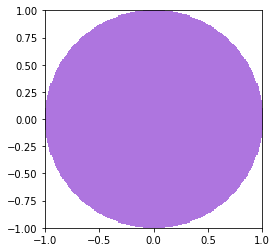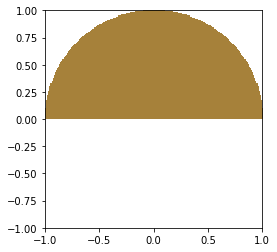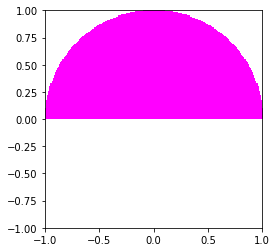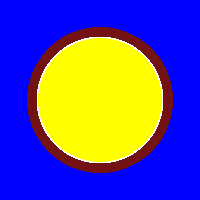# Modeling a Pin-Cell¶

pincell

This notebook is intended to demonstrate the basic features of the Python API for constructing input files and running OpenMC. In it, we will show how to create a basic reflective pin-cell model that is equivalent to modeling an infinite array of fuel pins. If you have never used OpenMC, this can serve as a good starting point to learn the Python API. We highly recommend having a copy of the Python API reference documentation open in another browser tab that you can refer to.

In :
%matplotlib inline
import openmc


## Defining Materials¶

Materials in OpenMC are defined as a set of nuclides with specified atom/weight fractions. To begin, we will create a material by making an instance of the Material class. In OpenMC, many objects, including materials, are identified by a "unique ID" that is simply just a positive integer. These IDs are used when exporting XML files that the solver reads in. They also appear in the output and can be used for identification. Since an integer ID is not very useful by itself, you can also give a material a name as well.

In :
uo2 = openmc.Material(1, "uo2")
print(uo2)

Material
ID             =	1
Name           =	uo2
Temperature    =	None
Density        =	None [sum]
S(a,b) Tables
Nuclides



On the XML side, you have no choice but to supply an ID. However, in the Python API, if you don't give an ID, one will be automatically generated for you:

In :
mat = openmc.Material()
print(mat)

Material
ID             =	2
Name           =
Temperature    =	None
Density        =	None [sum]
S(a,b) Tables
Nuclides



We see that an ID of 2 was automatically assigned. Let's now move on to adding nuclides to our uo2 material. The Material object has a method add_nuclide() whose first argument is the name of the nuclide and second argument is the atom or weight fraction.

In :
help(uo2.add_nuclide)

Help on method add_nuclide in module openmc.material:

add_nuclide(nuclide, percent, percent_type='ao') method of openmc.material.Material instance
Add a nuclide to the material

Parameters
----------
nuclide : str
percent : float
Atom or weight percent
percent_type : {'ao', 'wo'}
'ao' for atom percent and 'wo' for weight percent



We see that by default it assumes we want an atom fraction.

In :
# Add nuclides to uo2


Now we need to assign a total density to the material. We'll use the set_density for this.

In :
uo2.set_density('g/cm3', 10.0)


You may sometimes be given a material specification where all the nuclide densities are in units of atom/b-cm. In this case, you just want the density to be the sum of the constituents. In that case, you can simply run mat.set_density('sum').

With UO2 finished, let's now create materials for the clad and coolant. Note the use of add_element() for zirconium.

In :
zirconium = openmc.Material(2, "zirconium")
zirconium.set_density('g/cm3', 6.6)

water = openmc.Material(3, "h2o")
water.set_density('g/cm3', 1.0)

/home/romano/openmc/openmc/mixin.py:71: IDWarning: Another Material instance already exists with id=2.
warn(msg, IDWarning)


An astute observer might now point out that this water material we just created will only use free-atom cross sections. We need to tell it to use an $S(\alpha,\beta)$ table so that the bound atom cross section is used at thermal energies. To do this, there's an add_s_alpha_beta() method. Note the use of the GND-style name "c_H_in_H2O".

In :
water.add_s_alpha_beta('c_H_in_H2O')


When we go to run the transport solver in OpenMC, it is going to look for a materials.xml file. Thus far, we have only created objects in memory. To actually create a materials.xml file, we need to instantiate a Materials collection and export it to XML.

In :
mats = openmc.Materials([uo2, zirconium, water])


Note that Materials is actually a subclass of Python's built-in list, so we can use methods like append(), insert(), pop(), etc.

In :
mats = openmc.Materials()
mats.append(uo2)
mats += [zirconium, water]
isinstance(mats, list)

Out:
True

Finally, we can create the XML file with the export_to_xml() method. In a Jupyter notebook, we can run a shell command by putting ! before it, so in this case we are going to display the materials.xml file that we created.

In :
mats.export_to_xml()
!cat materials.xml

<?xml version='1.0' encoding='utf-8'?>
<materials>
<material depletable="true" id="1" name="uo2">
<density units="g/cm3" value="10.0" />
<nuclide ao="0.03" name="U235" />
<nuclide ao="0.97" name="U238" />
<nuclide ao="2.0" name="O16" />
</material>
<material id="2" name="zirconium">
<density units="g/cm3" value="6.6" />
<nuclide ao="0.5145" name="Zr90" />
<nuclide ao="0.1122" name="Zr91" />
<nuclide ao="0.1715" name="Zr92" />
<nuclide ao="0.1738" name="Zr94" />
<nuclide ao="0.028" name="Zr96" />
</material>
<material id="3" name="h2o">
<density units="g/cm3" value="1.0" />
<nuclide ao="2.0" name="H1" />
<nuclide ao="1.0" name="O16" />
<sab name="c_H_in_H2O" />
</material>
</materials>


### Element Expansion¶

Did you notice something really cool that happened to our Zr element? OpenMC automatically turned it into a list of nuclides when it exported it! The way this feature works is as follows:

• First, it checks whether Materials.cross_sections has been set, indicating the path to a cross_sections.xml file.
• If Materials.cross_sections isn't set, it looks for the OPENMC_CROSS_SECTIONS environment variable.
• If either of these are found, it scans the file to see what nuclides are actually available and will expand elements accordingly.

Let's see what happens if we change O16 in water to elemental O.

In :
water.remove_nuclide('O16')

mats.export_to_xml()
!cat materials.xml

<?xml version='1.0' encoding='utf-8'?>
<materials>
<material depletable="true" id="1" name="uo2">
<density units="g/cm3" value="10.0" />
<nuclide ao="0.03" name="U235" />
<nuclide ao="0.97" name="U238" />
<nuclide ao="2.0" name="O16" />
</material>
<material id="2" name="zirconium">
<density units="g/cm3" value="6.6" />
<nuclide ao="0.5145" name="Zr90" />
<nuclide ao="0.1122" name="Zr91" />
<nuclide ao="0.1715" name="Zr92" />
<nuclide ao="0.1738" name="Zr94" />
<nuclide ao="0.028" name="Zr96" />
</material>
<material id="3" name="h2o">
<density units="g/cm3" value="1.0" />
<nuclide ao="2.0" name="H1" />
<nuclide ao="0.999621" name="O16" />
<nuclide ao="0.000379" name="O17" />
<sab name="c_H_in_H2O" />
</material>
</materials>


We see that now O16 and O17 were automatically added. O18 is missing because our cross sections file (which is based on ENDF/B-VII.1) doesn't have O18. If OpenMC didn't know about the cross sections file, it would have assumed that all isotopes exist.

### The cross_sections.xml file¶

The cross_sections.xml tells OpenMC where it can find nuclide cross sections and $S(\alpha,\beta)$ tables. It serves the same purpose as MCNP's xsdir file and Serpent's xsdata file. As we mentioned, this can be set either by the OPENMC_CROSS_SECTIONS environment variable or the Materials.cross_sections attribute.

Let's have a look at what's inside this file:

In :
!cat $OPENMC_CROSS_SECTIONS | head -n 10 print(' ...') !cat$OPENMC_CROSS_SECTIONS | tail -n 10

<?xml version='1.0' encoding='utf-8'?>
<cross_sections>
<library materials="Ac225" path="Ac225.h5" type="neutron" />
<library materials="Ac226" path="Ac226.h5" type="neutron" />
<library materials="Ac227" path="Ac227.h5" type="neutron" />
<library materials="Ag107" path="Ag107.h5" type="neutron" />
<library materials="Ag109" path="Ag109.h5" type="neutron" />
<library materials="Ag110_m1" path="Ag110_m1.h5" type="neutron" />
<library materials="Ag111" path="Ag111.h5" type="neutron" />
<library materials="Al27" path="Al27.h5" type="neutron" />
...
<library materials="Cf253" path="wmp/098253.h5" type="wmp" />
<library materials="Cf254" path="wmp/098254.h5" type="wmp" />
<library materials="Es251" path="wmp/099251.h5" type="wmp" />
<library materials="Es252" path="wmp/099252.h5" type="wmp" />
<library materials="Es253" path="wmp/099253.h5" type="wmp" />
<library materials="Es254" path="wmp/099254.h5" type="wmp" />
<library materials="Es254_m1" path="wmp/099254m1.h5" type="wmp" />
<library materials="Es255" path="wmp/099255.h5" type="wmp" />
<library materials="Fm255" path="wmp/100255.h5" type="wmp" />
</cross_sections>


### Enrichment¶

Note that the add_element() method has a special argument enrichment that can be used for Uranium. For example, if we know that we want to create 3% enriched UO2, the following would work:

In :
uo2_three = openmc.Material()
uo2_three.set_density('g/cc', 10.0)


## Defining Geometry¶

At this point, we have three materials defined, exported to XML, and ready to be used in our model. To finish our model, we need to define the geometric arrangement of materials. OpenMC represents physical volumes using constructive solid geometry (CSG), also known as combinatorial geometry. The object that allows us to assign a material to a region of space is called a Cell (same concept in MCNP, for those familiar). In order to define a region that we can assign to a cell, we must first define surfaces which bound the region. A surface is a locus of zeros of a function of Cartesian coordinates $x$, $y$, and $z$, e.g.

• A plane perpendicular to the x axis: $x - x_0 = 0$
• A cylinder parallel to the z axis: $(x - x_0)^2 + (y - y_0)^2 - R^2 = 0$
• A sphere: $(x - x_0)^2 + (y - y_0)^2 + (z - z_0)^2 - R^2 = 0$

Between those three classes of surfaces (planes, cylinders, spheres), one can construct a wide variety of models. It is also possible to define cones and general second-order surfaces (tori are not currently supported).

Note that defining a surface is not sufficient to specify a volume -- in order to define an actual volume, one must reference the half-space of a surface. A surface half-space is the region whose points satisfy a positive or negative inequality of the surface equation. For example, for a sphere of radius one centered at the origin, the surface equation is $f(x,y,z) = x^2 + y^2 + z^2 - 1 = 0$. Thus, we say that the negative half-space of the sphere, is defined as the collection of points satisfying $f(x,y,z) < 0$, which one can reason is the inside of the sphere. Conversely, the positive half-space of the sphere would correspond to all points outside of the sphere.

Let's go ahead and create a sphere and confirm that what we've told you is true.

In :
sph = openmc.Sphere(r=1.0)


Note that by default the sphere is centered at the origin so we didn't have to supply x0, y0, or z0 arguments. Strictly speaking, we could have omitted R as well since it defaults to one. To get the negative or positive half-space, we simply need to apply the - or + unary operators, respectively.

(NOTE: Those unary operators are defined by special methods: __pos__ and __neg__ in this case).

In :
inside_sphere = -sph
outside_sphere = +sph


Now let's see if inside_sphere actually contains points inside the sphere:

In :
print((0,0,0) in inside_sphere, (0,0,2) in inside_sphere)
print((0,0,0) in outside_sphere, (0,0,2) in outside_sphere)

True False
False True


Everything works as expected! Now that we understand how to create half-spaces, we can create more complex volumes by combining half-spaces using Boolean operators: & (intersection), | (union), and ~ (complement). For example, let's say we want to define a region that is the top part of the sphere (all points inside the sphere that have $z > 0$.

In :
z_plane = openmc.ZPlane(z0=0)
northern_hemisphere = -sph & +z_plane


For many regions, OpenMC can automatically determine a bounding box. To get the bounding box, we use the bounding_box property of a region, which returns a tuple of the lower-left and upper-right Cartesian coordinates for the bounding box:

In :
northern_hemisphere.bounding_box

Out:
(array([-1., -1.,  0.]), array([1., 1., 1.]))

Now that we see how to create volumes, we can use them to create a cell.

In :
cell = openmc.Cell()
cell.region = northern_hemisphere

# or...
cell = openmc.Cell(region=northern_hemisphere)


By default, the cell is not filled by any material (void). In order to assign a material, we set the fill property of a Cell.

In :
cell.fill = water


### Universes and in-line plotting¶

A collection of cells is known as a universe (again, this will be familiar to MCNP/Serpent users) and can be used as a repeatable unit when creating a model. Although we don't need it yet, the benefit of creating a universe is that we can visualize our geometry while we're creating it.

In :
universe = openmc.Universe()

# this also works
universe = openmc.Universe(cells=[cell])


The Universe object has a plot method that will display our the universe as current constructed:

In :
universe.plot(width=(2.0, 2.0))

Out:
<matplotlib.image.AxesImage at 0x1548c7671cf8>By default, the plot will appear in the $x$-$y$ plane. We can change that with the basis argument.

In :
universe.plot(width=(2.0, 2.0), basis='xz')

Out:
<matplotlib.image.AxesImage at 0x1548c559c080>If we have particular fondness for, say, fuchsia, we can tell the plot() method to make our cell that color.

In :
universe.plot(width=(2.0, 2.0), basis='xz',
colors={cell: 'fuchsia'})

Out:
<matplotlib.image.AxesImage at 0x1548c5576c50>### Pin cell geometry¶

We now have enough knowledge to create our pin-cell. We need three surfaces to define the fuel and clad:

1. The outer surface of the fuel -- a cylinder parallel to the z axis
2. The inner surface of the clad -- same as above
3. The outer surface of the clad -- same as above

These three surfaces will all be instances of openmc.ZCylinder, each with a different radius according to the specification.

In :
fuel_or = openmc.ZCylinder(r=0.39)


With the surfaces created, we can now take advantage of the built-in operators on surfaces to create regions for the fuel, the gap, and the clad:

In :
fuel_region = -fuel_or


Now we can create corresponding cells that assign materials to these regions. As with materials, cells have unique IDs that are assigned either manually or automatically. Note that the gap cell doesn't have any material assigned (it is void by default).

In :
fuel = openmc.Cell(1, 'fuel')
fuel.fill = uo2
fuel.region = fuel_region

gap = openmc.Cell(2, 'air gap')
gap.region = gap_region


/home/romano/openmc/openmc/mixin.py:71: IDWarning: Another Cell instance already exists with id=1.
warn(msg, IDWarning)
/home/romano/openmc/openmc/mixin.py:71: IDWarning: Another Cell instance already exists with id=2.
warn(msg, IDWarning)


Finally, we need to handle the coolant outside of our fuel pin. To do this, we create x- and y-planes that bound the geometry.

In :
pitch = 1.26
left = openmc.XPlane(x0=-pitch/2, boundary_type='reflective')
right = openmc.XPlane(x0=pitch/2, boundary_type='reflective')
bottom = openmc.YPlane(y0=-pitch/2, boundary_type='reflective')
top = openmc.YPlane(y0=pitch/2, boundary_type='reflective')


The water region is going to be everything outside of the clad outer radius and within the box formed as the intersection of four half-spaces.

In :
water_region = +left & -right & +bottom & -top & +clad_or

moderator = openmc.Cell(4, 'moderator')
moderator.fill = water
moderator.region = water_region


OpenMC also includes a factory function that generates a rectangular prism that could have made our lives easier.

In :
box = openmc.rectangular_prism(width=pitch, height=pitch,
boundary_type='reflective')
type(box)

Out:
openmc.region.Intersection

Pay attention here -- the object that was returned is NOT a surface. It is actually the intersection of four surface half-spaces, just like we created manually before. Thus, we don't need to apply the unary operator (-box). Instead, we can directly combine it with +clad_or.

In :
water_region = box & +clad_or


The final step is to assign the cells we created to a universe and tell OpenMC that this universe is the "root" universe in our geometry. The Geometry is the final object that is actually exported to XML.

In :
root = openmc.Universe(cells=(fuel, gap, clad, moderator))

geom = openmc.Geometry()
geom.root_universe = root

# or...
geom = openmc.Geometry(root)
geom.export_to_xml()
!cat geometry.xml

<?xml version='1.0' encoding='utf-8'?>
<geometry>
<cell id="1" material="1" name="fuel" region="-3" universe="3" />
<cell id="2" material="void" name="air gap" region="3 -4" universe="3" />
<cell id="3" material="2" name="clad" region="4 -5" universe="3" />
<cell id="4" material="3" name="moderator" region="6 -7 8 -9 5" universe="3" />
<surface coeffs="0.0 0.0 0.39" id="3" type="z-cylinder" />
<surface coeffs="0.0 0.0 0.4" id="4" type="z-cylinder" />
<surface coeffs="0.0 0.0 0.46" id="5" type="z-cylinder" />
<surface boundary="reflective" coeffs="-0.63" id="6" type="x-plane" />
<surface boundary="reflective" coeffs="0.63" id="7" type="x-plane" />
<surface boundary="reflective" coeffs="-0.63" id="8" type="y-plane" />
<surface boundary="reflective" coeffs="0.63" id="9" type="y-plane" />
</geometry>


## Starting source and settings¶

The Python API has a module openmc.stats with various univariate and multivariate probability distributions. We can use these distributions to create a starting source using the openmc.Source object.

In :
point = openmc.stats.Point((0, 0, 0))
src = openmc.Source(space=point)


Now let's create a Settings object and give it the source we created along with specifying how many batches and particles we want to run.

In :
settings = openmc.Settings()
settings.source = src
settings.batches = 100
settings.inactive = 10
settings.particles = 1000

In :
settings.export_to_xml()
!cat settings.xml

<?xml version='1.0' encoding='utf-8'?>
<settings>
<run_mode>eigenvalue</run_mode>
<particles>1000</particles>
<batches>100</batches>
<inactive>10</inactive>
<source strength="1.0">
<space type="point">
<parameters>0 0 0</parameters>
</space>
</source>
</settings>


## User-defined tallies¶

We actually have all the required files needed to run a simulation. Before we do that though, let's give a quick example of how to create tallies. We will show how one would tally the total, fission, absorption, and (n,$\gamma$) reaction rates for $^{235}$U in the cell containing fuel. Recall that filters allow us to specify where in phase-space we want events to be tallied and scores tell us what we want to tally:

$$X = \underbrace{\int d\mathbf{r} \int d\mathbf{\Omega} \int dE}_{\text{filters}} \; \underbrace{f(\mathbf{r},\mathbf{\Omega},E)}_{\text{scores}} \psi (\mathbf{r},\mathbf{\Omega},E)$$

In this case, the where is "the fuel cell". So, we will create a cell filter specifying the fuel cell.

In :
cell_filter = openmc.CellFilter(fuel)

t = openmc.Tally(1)
t.filters = [cell_filter]


The what is the total, fission, absorption, and (n,$\gamma$) reaction rates in $^{235}$U. By default, if we only specify what reactions, it will gives us tallies over all nuclides. We can use the nuclides attribute to name specific nuclides we're interested in.

In :
t.nuclides = ['U235']
t.scores = ['total', 'fission', 'absorption', '(n,gamma)']


Similar to the other files, we need to create a Tallies collection and export it to XML.

In :
tallies = openmc.Tallies([t])
tallies.export_to_xml()
!cat tallies.xml

<?xml version='1.0' encoding='utf-8'?>
<tallies>
<filter id="1" type="cell">
<bins>1</bins>
</filter>
<tally id="1">
<filters>1</filters>
<nuclides>U235</nuclides>
<scores>total fission absorption (n,gamma)</scores>
</tally>
</tallies>


## Running OpenMC¶

Running OpenMC from Python can be done using the openmc.run() function. This function allows you to set the number of MPI processes and OpenMP threads, if need be.

In :
openmc.run()

                                %%%%%%%%%%%%%%%
%%%%%%%%%%%%%%%%%%%%%%%%
%%%%%%%%%%%%%%%%%%%%%%%%%%%%%%
%%%%%%%%%%%%%%%%%%%%%%%%%%%%%%%%%%
%%%%%%%%%%%%%%%%%%%%%%%%%%%%%%%%%%%%%%
%%%%%%%%%%%%%%%%%%%%%%%%%%%%%%%%%%%%%%%%
%%%%%%%%%%%%%%%%%%%%%%%%
%%%%%%%%%%%%%%%%%%%%%%%%
###############      %%%%%%%%%%%%%%%%%%%%%%%%
##################     %%%%%%%%%%%%%%%%%%%%%%%
###################     %%%%%%%%%%%%%%%%%%%%%%%
####################     %%%%%%%%%%%%%%%%%%%%%%
#####################     %%%%%%%%%%%%%%%%%%%%%
######################     %%%%%%%%%%%%%%%%%%%%
#######################     %%%%%%%%%%%%%%%%%%
#######################     %%%%%%%%%%%%%%%%%
######################     %%%%%%%%%%%%%%%%%
####################     %%%%%%%%%%%%%%%%%
#################     %%%%%%%%%%%%%%%%%
###############     %%%%%%%%%%%%%%%%
############     %%%%%%%%%%%%%%%
########     %%%%%%%%%%%%%%
%%%%%%%%%%%

| The OpenMC Monte Carlo Code
Copyright | 2011-2019 MIT and OpenMC contributors
Version | 0.11.0-dev
Git SHA1 | 61c911cffdae2406f9f4bc667a9a6954748bb70c
Date/Time | 2019-07-19 06:20:10

Maximum neutron transport energy: 20000000.000000 eV for U235
Writing summary.h5 file...
Initializing source particles...

====================>     K EIGENVALUE SIMULATION     <====================

Bat./Gen.      k            Average k
=========   ========   ====================
1/1    1.32572
2/1    1.46138
3/1    1.46068
4/1    1.39592
5/1    1.37519
6/1    1.38777
7/1    1.50242
8/1    1.42042
9/1    1.47458
10/1    1.49148
11/1    1.39339
12/1    1.40637    1.39988 +/- 0.00649
13/1    1.42972    1.40983 +/- 0.01063
14/1    1.46319    1.42317 +/- 0.01531
15/1    1.41538    1.42161 +/- 0.01196
16/1    1.38163    1.41494 +/- 0.01182
17/1    1.41257    1.41461 +/- 0.01000
18/1    1.43455    1.41710 +/- 0.00901
19/1    1.33136    1.40757 +/- 0.01241
20/1    1.41560    1.40837 +/- 0.01113
21/1    1.38911    1.40662 +/- 0.01021
22/1    1.28621    1.39659 +/- 0.01370
23/1    1.45693    1.40123 +/- 0.01343
24/1    1.46839    1.40603 +/- 0.01333
25/1    1.46738    1.41012 +/- 0.01306
26/1    1.43977    1.41197 +/- 0.01236
27/1    1.44066    1.41366 +/- 0.01173
28/1    1.39358    1.41254 +/- 0.01112
29/1    1.39142    1.41143 +/- 0.01057
30/1    1.38525    1.41012 +/- 0.01012
31/1    1.38025    1.40870 +/- 0.00973
32/1    1.45348    1.41074 +/- 0.00949
33/1    1.35893    1.40848 +/- 0.00935
34/1    1.32332    1.40493 +/- 0.00963
35/1    1.46285    1.40725 +/- 0.00952
36/1    1.33760    1.40457 +/- 0.00953
37/1    1.41117    1.40482 +/- 0.00917
38/1    1.45574    1.40664 +/- 0.00903
39/1    1.43472    1.40760 +/- 0.00876
40/1    1.30110    1.40405 +/- 0.00918
41/1    1.41765    1.40449 +/- 0.00889
42/1    1.45300    1.40601 +/- 0.00874
43/1    1.40491    1.40597 +/- 0.00847
44/1    1.42053    1.40640 +/- 0.00823
45/1    1.38805    1.40588 +/- 0.00801
46/1    1.34293    1.40413 +/- 0.00798
47/1    1.35441    1.40279 +/- 0.00787
48/1    1.29370    1.39991 +/- 0.00818
49/1    1.48467    1.40209 +/- 0.00826
50/1    1.41759    1.40248 +/- 0.00806
51/1    1.37151    1.40172 +/- 0.00790
52/1    1.42403    1.40225 +/- 0.00773
53/1    1.38826    1.40193 +/- 0.00755
54/1    1.48944    1.40392 +/- 0.00764
55/1    1.41452    1.40415 +/- 0.00747
56/1    1.47337    1.40566 +/- 0.00746
57/1    1.35700    1.40462 +/- 0.00738
58/1    1.40305    1.40459 +/- 0.00722
59/1    1.41608    1.40482 +/- 0.00708
60/1    1.47254    1.40618 +/- 0.00706
61/1    1.36847    1.40544 +/- 0.00696
62/1    1.34103    1.40420 +/- 0.00694
63/1    1.39510    1.40403 +/- 0.00681
64/1    1.40228    1.40399 +/- 0.00668
65/1    1.29401    1.40200 +/- 0.00686
66/1    1.42693    1.40244 +/- 0.00675
67/1    1.36447    1.40177 +/- 0.00666
68/1    1.37498    1.40131 +/- 0.00656
69/1    1.36958    1.40077 +/- 0.00647
70/1    1.38585    1.40053 +/- 0.00637
71/1    1.42133    1.40087 +/- 0.00627
72/1    1.44900    1.40164 +/- 0.00622
73/1    1.37696    1.40125 +/- 0.00613
74/1    1.48851    1.40261 +/- 0.00619
75/1    1.38933    1.40241 +/- 0.00610
76/1    1.41780    1.40264 +/- 0.00601
77/1    1.41054    1.40276 +/- 0.00592
78/1    1.38194    1.40246 +/- 0.00584
79/1    1.38446    1.40219 +/- 0.00576
80/1    1.37504    1.40181 +/- 0.00569
81/1    1.40550    1.40186 +/- 0.00561
82/1    1.49785    1.40319 +/- 0.00569
83/1    1.35613    1.40255 +/- 0.00565
84/1    1.41786    1.40275 +/- 0.00557
85/1    1.38444    1.40251 +/- 0.00550
86/1    1.40459    1.40254 +/- 0.00543
87/1    1.39923    1.40249 +/- 0.00536
88/1    1.44540    1.40304 +/- 0.00532
89/1    1.45962    1.40376 +/- 0.00530
90/1    1.37057    1.40335 +/- 0.00525
91/1    1.38115    1.40307 +/- 0.00519
92/1    1.35758    1.40252 +/- 0.00516
93/1    1.34508    1.40182 +/- 0.00514
94/1    1.31471    1.40079 +/- 0.00519
95/1    1.41434    1.40095 +/- 0.00513
96/1    1.33895    1.40023 +/- 0.00512
97/1    1.44716    1.40077 +/- 0.00509
98/1    1.38455    1.40058 +/- 0.00503
99/1    1.52127    1.40194 +/- 0.00516
100/1    1.35488    1.40141 +/- 0.00513
Creating state point statepoint.100.h5...

=======================>     TIMING STATISTICS     <=======================

Total time for initialization     = 7.5853e-01 seconds
Reading cross sections          = 7.3383e-01 seconds
Total time in simulation          = 6.5719e+00 seconds
Time in transport only          = 5.9772e+00 seconds
Time in inactive batches        = 4.8850e-01 seconds
Time in active batches          = 6.0834e+00 seconds
Time synchronizing fission bank = 5.9939e-03 seconds
Sampling source sites         = 5.1295e-03 seconds
SEND/RECV source sites        = 7.3640e-04 seconds
Time accumulating tallies       = 9.9301e-05 seconds
Total time for finalization       = 1.1585e-04 seconds
Total time elapsed                = 7.3346e+00 seconds
Calculation Rate (inactive)       = 20471.0 particles/second
Calculation Rate (active)         = 14794.4 particles/second

============================>     RESULTS     <============================

k-effective (Collision)     = 1.39737 +/- 0.00470
k-effective (Track-length)  = 1.40141 +/- 0.00513
k-effective (Absorption)    = 1.39596 +/- 0.00308
Combined k-effective        = 1.39719 +/- 0.00286
Leakage Fraction            = 0.00000 +/- 0.00000



Great! OpenMC already told us our k-effective. It also spit out a file called tallies.out that shows our tallies. This is a very basic method to look at tally data; for more sophisticated methods, see other example notebooks.

In :
!cat tallies.out

 ============================>     TALLY 1     <============================

Cell 1
U235
Total Reaction Rate                  0.731003 +/- 0.00253759
Fission Rate                         0.547587 +/- 0.00210114
Absorption Rate                      0.657406 +/- 0.0024539
(n,gamma)                            0.109821 +/- 0.000368054


## Geometry plotting¶

We saw before that we could call the Universe.plot() method to show a universe while we were creating our geometry. There is also a built-in plotter in the Fortran codebase that is much faster than the Python plotter and has more options. The interface looks somewhat similar to the Universe.plot() method. Instead though, we create Plot instances, assign them to a Plots collection, export it to XML, and then run OpenMC in geometry plotting mode. As an example, let's specify that we want the plot to be colored by material (rather than by cell) and we assign yellow to fuel and blue to water.

In :
p = openmc.Plot()
p.filename = 'pinplot'
p.width = (pitch, pitch)
p.pixels = (200, 200)
p.color_by = 'material'
p.colors = {uo2: 'yellow', water: 'blue'}


With our plot created, we need to add it to a Plots collection which can be exported to XML.

In :
plots = openmc.Plots([p])
plots.export_to_xml()
!cat plots.xml

<?xml version='1.0' encoding='utf-8'?>
<plots>
<plot basis="xy" color_by="material" filename="pinplot" id="1" type="slice">
<origin>0.0 0.0 0.0</origin>
<width>1.26 1.26</width>
<pixels>200 200</pixels>
<color id="1" rgb="255 255 0" />
<color id="3" rgb="0 0 255" />
</plot>
</plots>


Now we can run OpenMC in plotting mode by calling the plot_geometry() function. Under the hood this is calling openmc --plot.

In :
openmc.plot_geometry()

                                %%%%%%%%%%%%%%%
%%%%%%%%%%%%%%%%%%%%%%%%
%%%%%%%%%%%%%%%%%%%%%%%%%%%%%%
%%%%%%%%%%%%%%%%%%%%%%%%%%%%%%%%%%
%%%%%%%%%%%%%%%%%%%%%%%%%%%%%%%%%%%%%%
%%%%%%%%%%%%%%%%%%%%%%%%%%%%%%%%%%%%%%%%
%%%%%%%%%%%%%%%%%%%%%%%%
%%%%%%%%%%%%%%%%%%%%%%%%
###############      %%%%%%%%%%%%%%%%%%%%%%%%
##################     %%%%%%%%%%%%%%%%%%%%%%%
###################     %%%%%%%%%%%%%%%%%%%%%%%
####################     %%%%%%%%%%%%%%%%%%%%%%
#####################     %%%%%%%%%%%%%%%%%%%%%
######################     %%%%%%%%%%%%%%%%%%%%
#######################     %%%%%%%%%%%%%%%%%%
#######################     %%%%%%%%%%%%%%%%%
######################     %%%%%%%%%%%%%%%%%
####################     %%%%%%%%%%%%%%%%%
#################     %%%%%%%%%%%%%%%%%
###############     %%%%%%%%%%%%%%%%
############     %%%%%%%%%%%%%%%
########     %%%%%%%%%%%%%%
%%%%%%%%%%%

| The OpenMC Monte Carlo Code
Copyright | 2011-2019 MIT and OpenMC contributors
Version | 0.11.0-dev
Git SHA1 | 61c911cffdae2406f9f4bc667a9a6954748bb70c
Date/Time | 2019-07-19 06:20:18

=======================>     PLOTTING SUMMARY     <========================

Plot ID: 1
Plot file: pinplot.ppm
Universe depth: -1
Plot Type: Slice
Origin: 0 0 0
Width: 1.26 1.26
Coloring: Materials
Basis: XY
Pixels: 200 200

Processing plot 1: pinplot.ppm...


OpenMC writes out a peculiar image with a .ppm extension. If you have ImageMagick installed, this can be converted into a more normal .png file.

In :
!convert pinplot.ppm pinplot.png


We can use functionality from IPython to display the image inline in our notebook:

In :
from IPython.display import Image
Image("pinplot.png")

Out:That was a little bit cumbersome. Thankfully, OpenMC provides us with a method on the Plot class that does all that "boilerplate" work.

In :
p.to_ipython_image()

Out: